# Stock Market Technical Indicators

Using The Python Programming Language

Disclaimer: The material in this article is purely educational and should not be taken as professional investment advice. Invest at your own discretion.

# What Are Stock Market Technical Indicators ?

Stock market technical indicators are signals used to interpret stock or financial data trends to attempt to predict future price movements within the market. Stock indicators help investors to make trading decisions.

# Types of Technical Indicators

Simple Moving Average (SMA): A simple moving average is a technical trend indicator that can aid in determining if an asset price will continue or if it will reverse a bull or bear trend. A simple moving average can be enhanced as an exponential moving average (EMA) that is more heavily weighted on recent price action. -investopedia

Exponential Moving Average (EMA): The EMA is a moving average that places a greater weight and significance on the most recent data points. Like all moving averages, this technical trend indicator is used to produce buy and sell signals based on crossovers and divergences from the historical average. -investopedia

Moving Average Convergence Divergence (MACD) : Moving Average Convergence Divergence (MACD) is a trend-following momentum indicator that shows the relationship between two moving averages of a security’s price. The MACD is calculated by subtracting the 26-period Exponential Moving Average (EMA) from the 12-period EMA. -investopedia

Relative Strength Index (RSI): The relative strength index (RSI) is a momentum indicator used in technical analysis that measures the magnitude of recent price changes to evaluate overbought or oversold conditions in the price of a stock or other asset. -investopedia

These are the indicators that we will be programming in this article using python.

# Some Trading Strategies Using The Indicators

Moving Average Crossover: In statistics and stock market technical analysis, a moving average crossover happens when two moving averages cross one another. There are many different types of moving averages for example Simple Moving Averages (SMA), Exponential Moving Averages, Smoothed Moving Averages (SMMA) , and Weighted Moving Average (LWMA). In this article I will show you how to program a dual simple moving average crossover strategy and a three exponential moving average crossover strategy.

In the dual moving average crossover trading strategy, these crossovers are points of decision to buy or sell an asset. The Technical Approach suggests that when the Short Term Moving Average (STMA) moves above the Long Term Moving Average (LTMA), that represents a Buy (or Long) signal and when the LTMA moves above the STMA, that represents a Sell (or short) signal.

Buy: STMA > LTMA
Sell: STMA < LTMA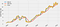Dual Simple Moving Average Crossover Chart

The three exponential moving average crossover strategy, is an approach to trading that uses 3 exponential moving averages of various lengths. The strategy says buy when the middle moving average crosses above the long/slow moving average and the short/fast moving average crosses above the middle moving average. The strategy also tells us to sell if the short/fast moving average crosses below the middle moving average.

Buy: Middle > Long
Sell: Short > Middle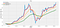Three Moving Average Crossover Chart

Moving Average Convergence /Divergence (MACD) Crossover : MACD, short for moving average convergence/divergence, is a trading indicator used in technical analysis of stock prices. When the MACD indicator crosses the signal line this indicates a momentum shift in the stock price. For example if the MACD indicator is greater than the signal line this is considered a bullish crossover and indicates a good time to buy, and when the MACD indicator is less than the signal line, this is considered a bearish crossover and indicates a good time to sell.

Buy: MACD > Signal Line
Sell: MACD < Signal Line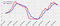MACD Crossover Chart

Relative Strength Index (RSI): RSI is a technical indicator, which is used in the analysis of financial markets to determine if a stock is being over bought or over sold. A common time period to use for RSI is 14 days. The RSI returns values on a scale from 0 to 100, with high and low level values marked at (70 and 30), (80 and 20 ), and (90 and 10). The higher the high level and the lower the low level indicate a stronger price momentum shift. For example RSI is considered overbought when above 70 and oversold when below 30. RSI values of 50 represent a neutral condition.

Sell: RSI = 70 or greater
Buy: RSI = 30 or lower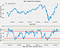RSI Chart

If you prefer not to read this article and would like a video representation of it, you can check out the YouTube Video . It goes through everything in this article with a little more detail, and will help make it easy for you to start programming even if you don’t have the programming language Python installed on your computer. Or you can use both as supplementary materials for learning !

# Programming

First, I want to create a description about the program so that I can simply read the description and know what the program is supposed to do or what the program is about.

`#Description: Create and Plot Multiple Technical Indicators`

Import the libraries that we will need throughout the program.

`#Import the librariesimport numpy as npimport pandas as pdimport matplotlib.pyplot as pltplt.style.use('fivethirtyeight')`

Next, load the stock data. I loaded data for Tesla (TSLA) with data from Nov 25, 2019 to Nov 23, 2020. Since I am using Google’s website, I need to use it’s library to upload the data.

`#Load the datafrom google.colab import files # Use to load data on Google Colabfiles.upload() # Use to load data on Google Colab`

Let’s store the data into a data frame and change the indices from integers to dates. Then show the data set.

`#Store the data (The data is from 2019-Nov-25 to 2020-Nov-23)df = pd.read_csv('TSLA_Stock_Data.csv')#Set the date as the indexdf = df.set_index(pd.DatetimeIndex(df['Date'].values))#Show the datadf`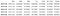Sample of the TSLA stock data

Create functions to calculate the Simple Moving Average (SMA) and the Exponential Moving Average (EMA).

`#Create functions to calculate the SMA, & the EMA#Create the Simple Moving Average Indicator#Typical time periods for moving averages are 15, 20,& 30#Create the Simple Moving Average Indicatordef SMA(data, period=30, column='Close'):  return data[column].rolling(window=period).mean()#Create the Exponential Moving Average Indicatordef EMA(data, period=20, column='Close'):  return data[column].ewm(span=period, adjust=False).mean()`

Now let’s create a function to calculate the Moving Average Convergence/Divergence (MACD) indicator. The recommended periods are 26 days for the long period EMA, 12 days for the short period EMA, and 9 days for the signal line EMA.

`def MACD(data, period_long=26, period_short=12, period_signal=9, column='Close'):    #Calculate the Short Term Exponential Moving Average    ShortEMA = EMA(data, period_short, column=column) #AKA Fast moving average    #Calculate the Long Term Exponential Moving Average    LongEMA = EMA(data, period_long, column=column) #AKA Slow moving average    #Calculate the Moving Average Convergence/Divergence (MACD)    data['MACD'] = ShortEMA - LongEMA    #Calcualte the signal line    data['Signal_Line'] = EMA(data, period_signal, column='MACD')#data['MACD'].ewm(span=period_signal, adjust=False).mean()            return data`

The last indicator that I will create a function for is the Relative Strength Index (RSI) indicator with a common time period of 14 days.

`#Create RSI functiondef RSI(data, period = 14, column = 'Close'):  delta = data[column].diff(1) #Use diff() function to find the discrete difference over the column axis with period value equal to 1  delta = delta.dropna() # or delta[1:]  up =  delta.copy() #Make a copy of this object’s indices and data  down = delta.copy() #Make a copy of this object’s indices and data  up[up < 0] = 0   down[down > 0] = 0   data['up'] = up  data['down'] = down  AVG_Gain = SMA(data, period, column='up')#up.rolling(window=period).mean()  AVG_Loss = abs(SMA(data, period, column='down'))#abs(down.rolling(window=period).mean())  RS = AVG_Gain / AVG_Loss  RSI = 100.0 - (100.0/ (1.0 + RS))    data['RSI'] = RSI  return data`

Now time to add these indicators to the data set and show them !

`#Creating the data set MACD(df)RSI(df)df['SMA'] = SMA(df)df['EMA'] = EMA(df)#Show the datadf`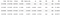Sample of the data set with the indicators

Time to plot the indicators on a chart. First I will plot the MACD indicator and the signal line.

`#Plot the chart#Create a list of columns to keepcolumn_list = ['MACD','Signal_Line']df[column_list].plot(figsize=(12.2,6.4)) #Plot the dataplt.title('MACD for TSLA')plt.show();`

Plot the Simple Moving Average (SMA)indicator.

`#Plot the chart#Create a list of columns to keepcolumn_list = ['SMA','Close']df[column_list].plot(figsize=(12.2,6.4)) #Plot the dataplt.title('SMA for TSLA')plt.ylabel('USD Price (\$)')plt.show();`

Plot the Exponential Moving Average (EMA) indicator.

`#Plot the chart#Create a list of columns to keepcolumn_list = ['EMA','Close']df[column_list].plot(figsize=(12.2,6.4)) #Plot the dataplt.title('EMA for TSLA')plt.ylabel('USD Price (\$)')plt.show();`

Last but not least plot the Relative Strength Index (RSI) indicator.

`#Plot the chart#Create a list of columns to keep#Sell: RSI = 70 or greater#Buy: RSI = 30 or lowercolumn_list = ['RSI']df[column_list].plot(figsize=(12.2,6.4)) #Plot the dataplt.title('RSI for TSLA')plt.show();`

Now, with these functions and charts you can create your own trading strategy! Keep in mind that redundancy is a common problem in technical analysis that occurs when the same types of indicators are applied to one chart, and redundant signals can be misleading although some traders intentionally apply multiple indicators of the same type, in the hopes of finding confirmation for an expected price move.

With that being said they some indicators can be complementary to each other, for example moving average crossovers can be complementary to RSI to confirm RSI indications that a market is overbought or oversold. That’s it, we are done creating this program !If you want to start an investment portfolio, then sign up with WeBull using this link and get FREE stocks just for opening an account and funding it with an initial deposit of \$100 or more! It’s free stocks that you can either sell, play with or create your own trading strategy with. For a free stock trading app, I highly recommend it.

If you are interested in reading more on algorithmic trading then you might want to read Hands-On Machine Learning for Algorithmic Trading: Design and implement investment strategies based on smart algorithms that learn from data using Python. The book will show you how to implement machine learning algorithms to build, train, and validate algorithmic models. It will also show you how to create your own algorithmic design process to apply probabilistic machine learning approaches to trading decisions, and the book will show you how to develop neural networks for algorithmic trading to perform time series forecasting and smart analytics.

Thanks for reading this article I hope it’s helpful to you all! If you enjoyed this article and found it helpful please leave some claps to show your appreciation. Keep up the learning, and if you like machine learning, mathematics, computer science, programming or algorithm analysis, please visit and subscribe to my YouTube channels (randerson112358 & compsci112358 ).

## More from randerson112358

A programmer that loves Computer Science: https://www.youtube.com/user/randerson112358 https://www.youtube.com/channel/UCbmb5IoBtHZTpYZCDBOC1